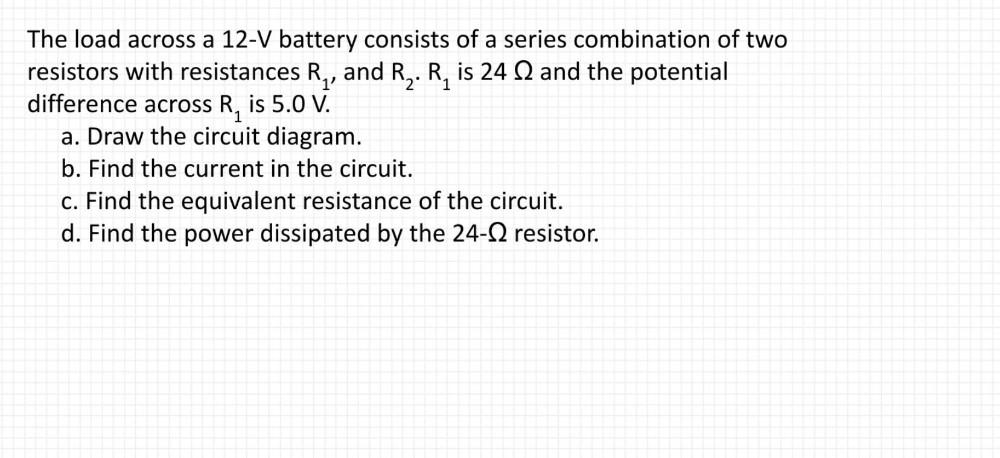Question:

# The load across a 12-V battery consists of a series combination of two resistors with resistances R₁ and R₂ . R₁ is 24Ω and the potential difference across R₁ is 5.0 V. (A)Draw the circuit diagram. (BThe load across a 12-V battery consists of a series combination of two resistors with resistances R₁ and R₂ . R₁ is 24Ω and the potential difference across R₁ is 5.0 V. (A)Draw the circuit diagram. (B) Find the current in the circuit. (C) Find the equivalent resistance of the circuit. (D) Find the power dissipated by the 24-Ω resistor.